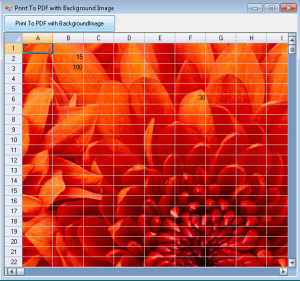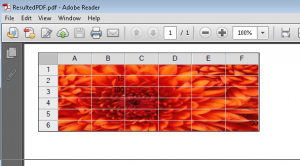Spread for .Net control support printing SpreadSheet to a Portable Document Format i.e. PDF file using the PrintToPdf method in the PrintInfo class. Recently one of our customer asked if we can print the background image while printing a PDF file from Spread. Although it is not directly supported in Spread but here in this blog lets discuss a workaround for this. The approach is simple. Set the transparent backcolor in Spread so that the image is visible behind the sheet. And since Spread's PrintBackground event occurs before each page is printed hence draw background image in Spread control. Following code explains the above implementation :

{
System.Drawing.Drawing2D.GraphicsState saveState = e.Graphics.Save();
Rectangle rect = e.SheetRectangle;
e.Graphics.SetClip(rect);
e.Graphics.SetClip(rect);
e.Graphics.Restore(saveState);
}
private float AdjustWorkaroundForPDFPrint(float value)
{
float _ptperInch = 72;
//Points Per inch
float pixelPointFactor = (float)(_ptperInch / 96);
//pixel point factor base on graphic dpi
float displayPointFactor = (float)(_ptperInch / 100);
//point factor base on display graphic unit
return (float)(value * displayPointFactor / pixelPointFactor);
}Spread With BackGround ImageExported PDF Please refer to the sample for detailed implementation of the same. DownloadSample_CS DownloadSample_VB